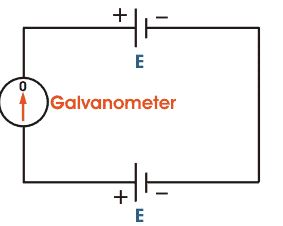# Potentiometer

A potentiometer is an instrument for measuring the potential (voltage) in a circuit. Before the introduction of the moving coil and digital volt meters, potentiometers were used in measuring voltage. Potentiometer is an accurate instruments used to compare emf's of a cells,Potential difference between two points of the electric wire.Potentiometer is based on the principle that potential drop across any portion of th wire of uniform crossection is proportional to the length of that portion of thw wire when a constant current flows through the wire .Here in the figure it is clear that if the voltage of both battery cells is exactly equal, there will be no circulating current in the circuit and hence the galvanometer shows null deflection. The working principle of potentiometer depends upon this phenomenon.